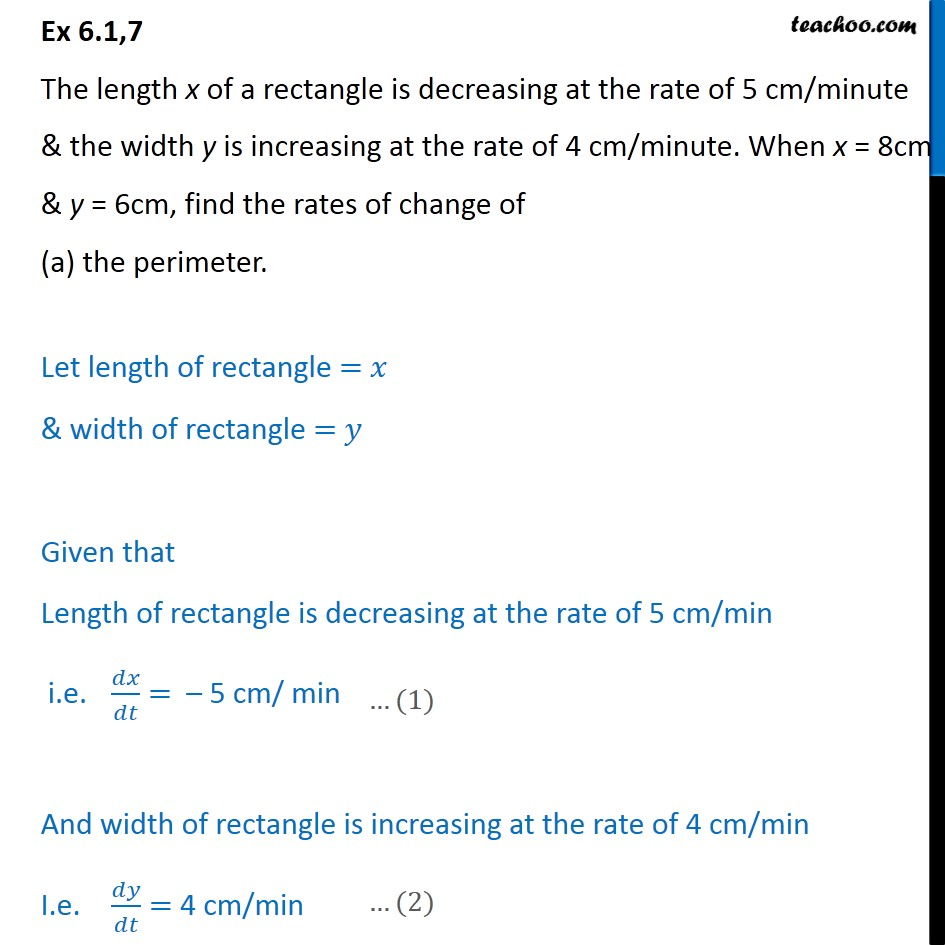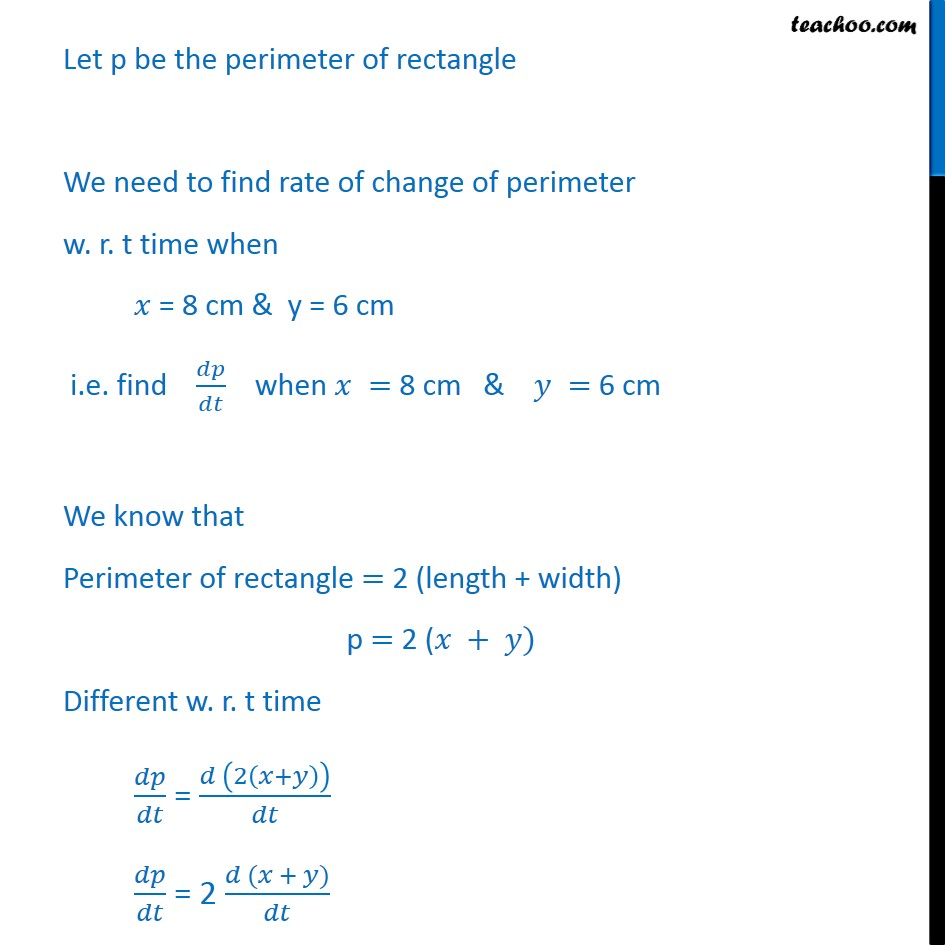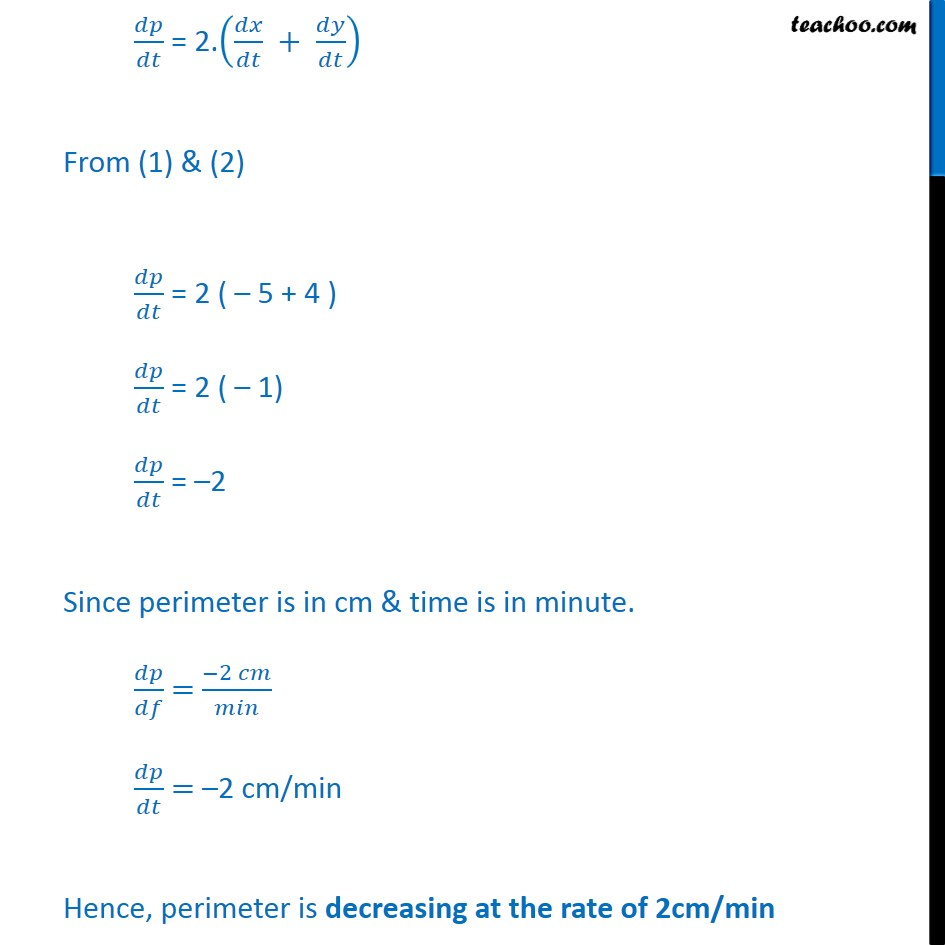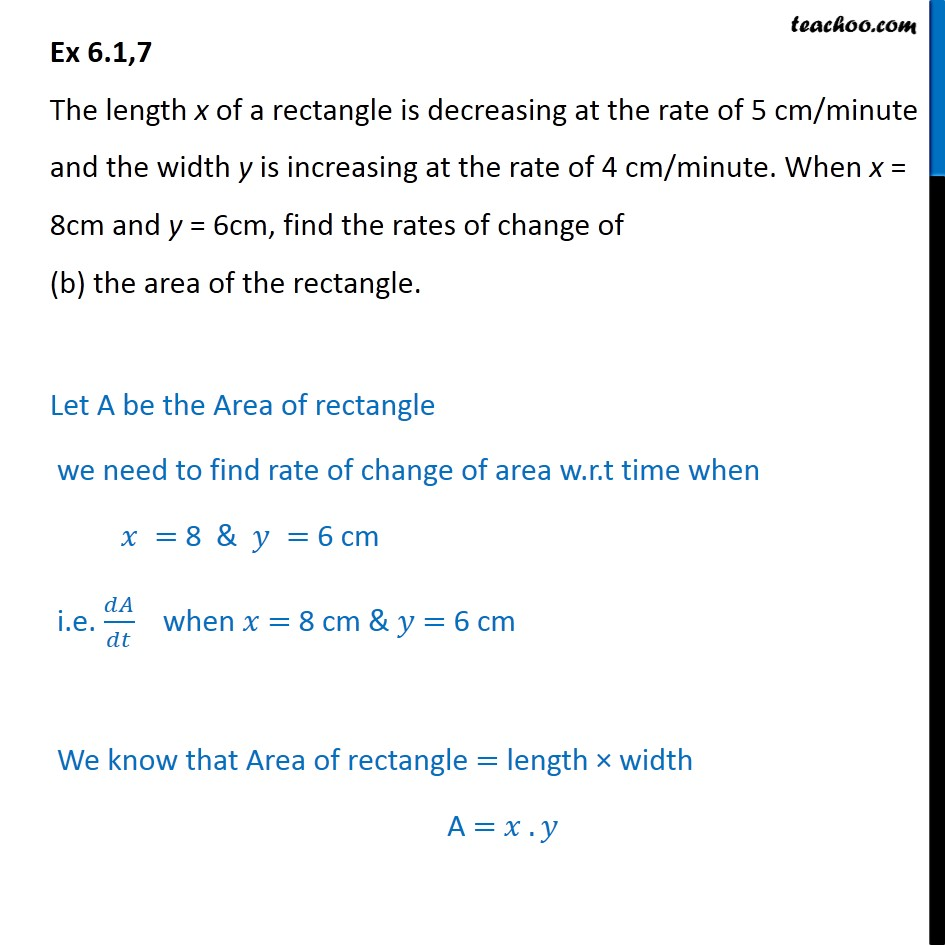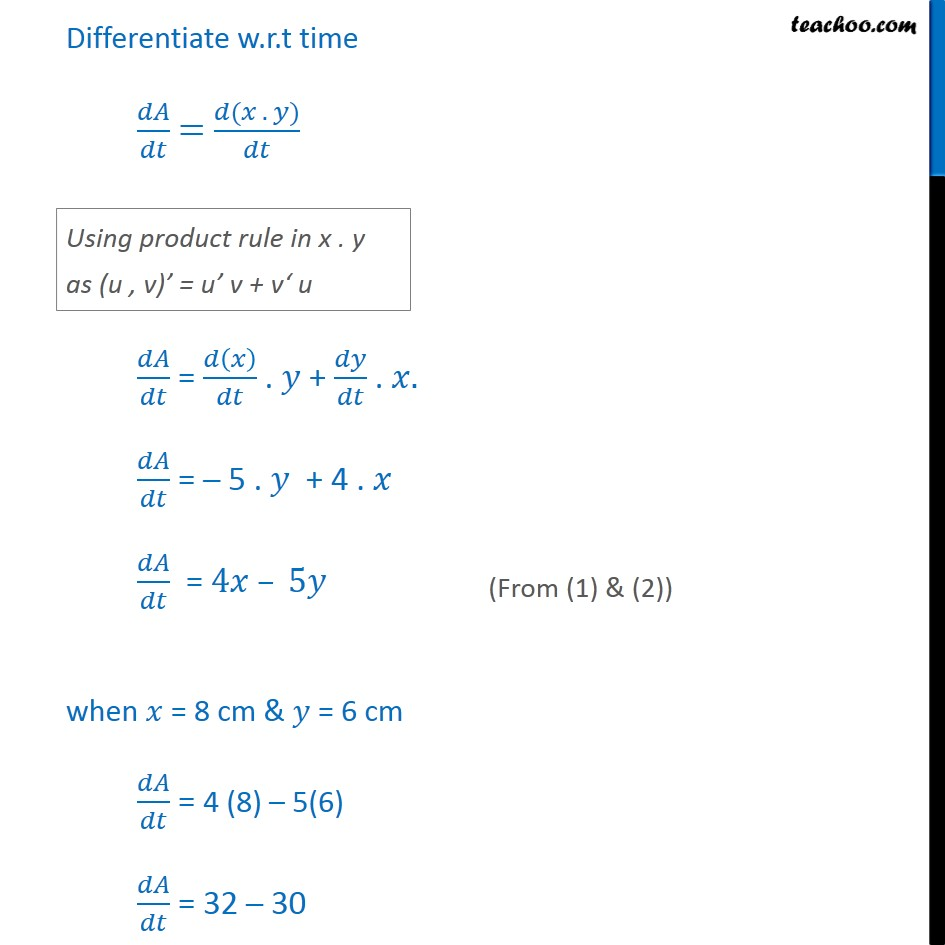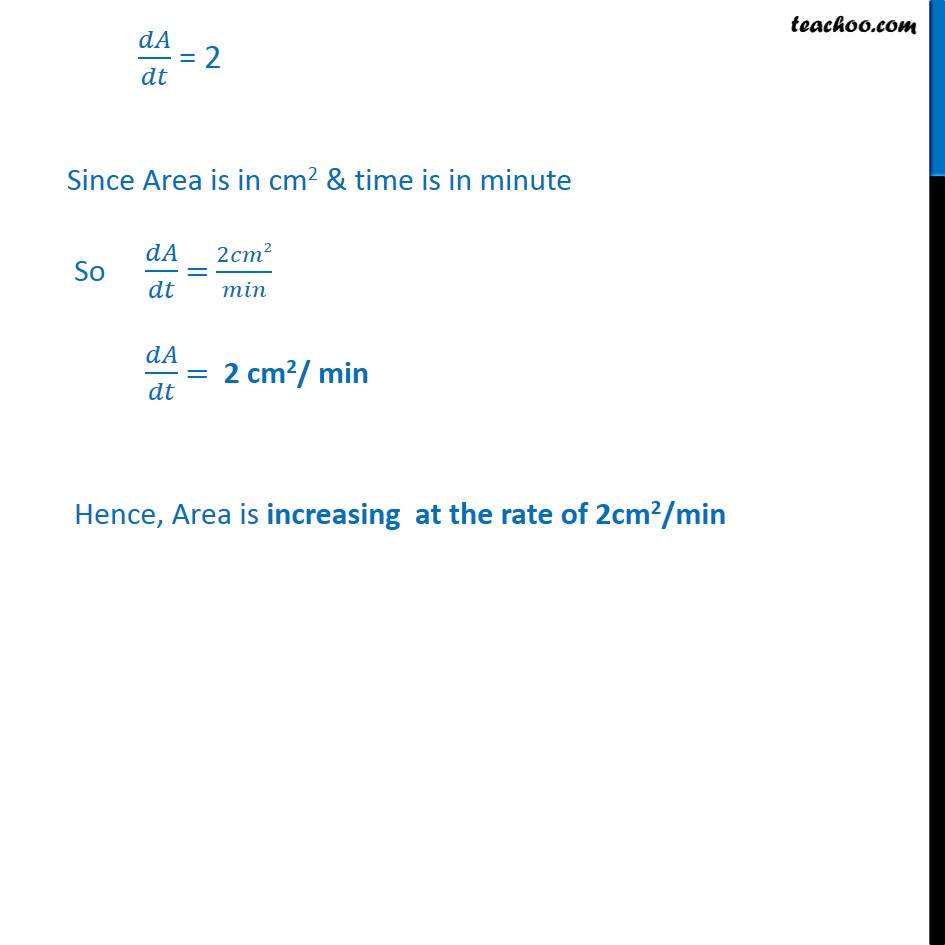1. Chapter 6 Class 12 Application of Derivatives
2. Concept wise
3. Finding rate of change

Transcript

Ex 6.1,7 The length x of a rectangle is decreasing at the rate of 5 cm/minute & the width y is increasing at the rate of 4 cm/minute. When x = 8cm & y = 6cm, find the rates of change of (a) the perimeter. Let length of rectangle = 𝑥 & width of rectangle = 𝑦 Given that Length of rectangle is decreasing at the rate of 5 cm/min i.e. 𝑑𝑥/𝑑𝑡 = – 5 cm/ min And width of rectangle is increasing at the rate of 4 cm/min I.e. 𝑑𝑦/𝑑𝑡 = 4 cm/min Let p be the perimeter of rectangle We need to find rate of change of perimeter w. r. t time when 𝑥 = 8 cm & y = 6 cm i.e. find 𝑑𝑝/𝑑𝑡 when 𝑥 = 8 cm & 𝑦 = 6 cm We know that Perimeter of rectangle = 2 (length + width) p = 2 (𝑥 + 𝑦) Different w. r. t time 𝑑𝑝/𝑑𝑡 = (𝑑 (2(𝑥+𝑦)))/𝑑𝑡 𝑑𝑝/𝑑𝑡 = 2 (𝑑 (𝑥 + 𝑦))/𝑑𝑡 𝑑𝑝/𝑑𝑡 = 2.(𝑑𝑥/𝑑𝑡 + 𝑑𝑦/𝑑𝑡) From (1) & (2) 𝑑𝑝/𝑑𝑡 = 2 ( – 5 + 4 ) 𝑑𝑝/𝑑𝑡 = 2 ( – 1) 𝑑𝑝/𝑑𝑡 = –2 Since perimeter is in cm & time is in minute. 𝑑𝑝/𝑑𝑓 = (−2 𝑐𝑚)/𝑚𝑖𝑛 𝑑𝑝/𝑑𝑡 = –2 cm/min Hence, perimeter is decreasing at the rate of 2cm/min Ex 6.1,7 The length x of a rectangle is decreasing at the rate of 5 cm/minute and the width y is increasing at the rate of 4 cm/minute. When x = 8cm and y = 6cm, find the rates of change of (b) the area of the rectangle. Let A be the Area of rectangle we need to find rate of change of area w.r.t time when 𝑥 = 8 & 𝑦 = 6 cm i.e. 𝑑𝐴/𝑑𝑡 when 𝑥 = 8 cm & 𝑦 = 6 cm We know that Area of rectangle = length × width A = 𝑥 . 𝑦 Differentiate w.r.t time 𝑑𝐴/𝑑𝑡 = (𝑑(𝑥 . 𝑦))/𝑑𝑡 Using product rule in x . y as (u , v)’ = u’ v + v‘ u 𝑑𝐴/𝑑𝑡 = 𝑑(𝑥)/𝑑𝑡 . 𝑦 + 𝑑𝑦/𝑑𝑡 . 𝑥. 𝑑𝐴/𝑑𝑡 = – 5 . 𝑦 + 4 . 𝑥 𝑑𝐴/𝑑𝑡 = 4𝑥 – 5𝑦 when 𝑥 = 8 cm & 𝑦 = 6 cm 𝑑𝐴/𝑑𝑡 = 4 (8) – 5(6) 𝑑𝐴/𝑑𝑡 = 32 – 30 𝑑𝐴/𝑑𝑡 = 2 Since Area is in cm2 & time is in minute So 𝑑𝐴/𝑑𝑡 = 2𝑐𝑚2/𝑚𝑖𝑛 𝑑𝐴/𝑑𝑡 = 2 cm2/ min Hence, Area is increasing at the rate of 2cm2/min

Finding rate of change

About the AuthorDavneet Singh
Davneet Singh is a graduate from Indian Institute of Technology, Kanpur. He has been teaching from the past 9 years. He provides courses for Maths and Science at Teachoo.